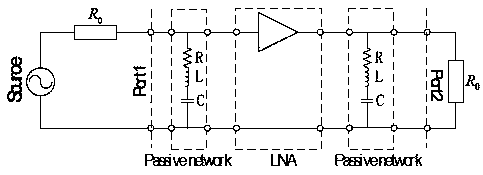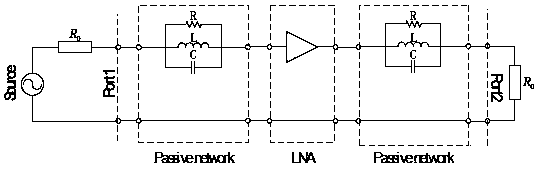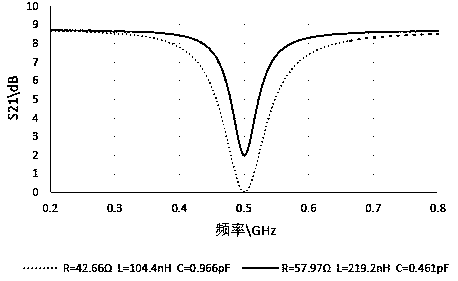# A negative group delay circuit based on RLC and a low noise amplifier and a design method thereof

## A low-noise amplifier and negative group delay technology, which is applied to electrical components, impedance networks, multi-terminal pair networks, etc.

Inactive Publication Date: 2018-12-28
NANJING UNIV OF INFORMATION SCI & TECH
0 Cites 3 Cited by

## AI-Extracted Technical Summary

### Problems solved by technology

However, the current research results of negative group delay circuits are mainly concentrated in western d...
View more

## Abstract

The invention relates to a negative group delay circuit based on RLC and low noise amplifier and a design method thereof, the circuit includes a signal source, the signal source is connected with a network input port, the network input port is connected with the network output port, the network output port is externally connected with a load, the impedance of the signal source and the load impedance are both R0, two groups of resonant circuits based on RLC are arranged between the network input port and the network output port, and a low noise amplifier is connected between the two groups of resonant circuits based on RLC. The group delay of the circuit can be set according to one's own needs, and the loss parameters can be inserted into the circuit to calculate the required circuit parameters, such as the values of resistance R, inductance L and capacitance C in the circuit. And the insertion loss of the circuit can be zero, which can compensate the loss caused by RLC negative group delay circuit by LNA to some extent, and improve the NGD bandwidth.

Application Domain

Multiple-port networks

Technology Topic

CapacitanceEngineering +7

## Image

•••## Examples

• Experimental program(1)

### Example Embodiment

 The present invention will now be described in further detail with reference to the drawings.
 Such as figure 1 As shown, a negative group delay circuit based on a series RLC and a low noise amplifier is characterized in that it includes a signal source, the signal source is connected to a network input port, and the network input port is connected to a network output port, The network output port is connected to an external load, and the impedance of the signal source and the load impedance are both R 0 Two sets of RLC-based resonant circuits are arranged between the network input port and the network output port, and a low noise amplifier is connected between the two sets of RLC-based resonant circuits. The RLC-based resonant circuit is composed of resistors R, The inductor L and the capacitor C are formed in series in sequence, and the RLC-based resonance circuit is connected in parallel between the network input port and the network output port.
 A design method of negative group delay circuit based on series RLC and low noise amplifier, which is characterized in that: according to the S parameter theory, the S parameter matrix of the group delay circuit is derived, and the insertion loss in the derived S parameter matrix is ​​used , By the formula To find the phase function of the circuit, and finally defined by the group delay To find the group delay function, at the same time clarify the reflection coefficient and gain of the low noise amplifier LNA, the bandwidth of the group delay circuit, and then comprehensively calculate the parameters of the NGD circuit according to the specified negative group delay, gain, NGD bandwidth and matching level For resistance R, inductance L, and capacitance C, the specific steps are as follows:
 First, define the S parameter model of the low noise amplifier LNA:

 Where r and t are the reflection coefficient and insertion gain of the LNA, respectively; in this embodiment, t=8.5dB, r=-22dB, and LNA selects LEE-9+.
 According to the S parameter theory,
 will Bring in in,
 Derive the S parameters of the circuit:

 Where R 0 Is the characteristic impedance connected to the input and output ports of the network; in this embodiment, R 0 =50Ω;
 The capacitance C in the circuit satisfies:

 Where ω 0 Is the center frequency of the circuit;
 At center frequency ω 0 The S parameters at time are as follows:

 According to the circuit system theory, set jω as the angular frequency of the circuit, and the group delay formula is:

 among them
 From formulas (2), (3), (5), (6), we can get at the center frequency ω 0 Group delay at:

 When ω=ω 0 When, by formula (4):

 make
 From formulas (7), (8), (9):


 According to formulas (3), (10), (11), any group delay τ can be set 0 , Insert the gain g, set the known t=8.5dB, r=-22dB, R 0 =50Ω bring in, and then obtain the resistance R, the inductance L, and the capacitance C.
 When the group delay is set to -4ns and the insertion gain is 0dB, the calculated result is that the resistance R=42.66Ω, the inductance L=104.4nH, and the capacitance C=0.966pF.
 When the group delay is set to -5ns and the insertion gain is 2dB, the calculated result is that the resistance R=57.97Ω, the inductance L=219.2nH, and the capacitance C=0.461pF.
 Such as image 3 As shown, when the center frequency is 0.5GHz, when the resistance R=42.66Ω, the inductance L=104.4nH, and the capacitance C=0.966pF, the simulation can obtain an insertion gain of 0dB.
 Such as image 3 As shown, when the center frequency is 0.5GHz, when the resistance R=57.97Ω, the inductance L=219.2nH, and the capacitance C=0.461pF, the simulation can obtain an insertion gain of 2dB.
 Such as Figure 4 As shown, when the center frequency is 0.5GHz, when the resistance R=42.66Ω, the inductance L=104.4nH, and the capacitance C=0.966pF, the simulation can obtain a group delay of -4ns.
 Such as Figure 4 As shown, when the center frequency is 0.5GHz, when the resistance R=57.97Ω, the inductance L=219.2nH, and the capacitance C=0.461pF, the simulation can obtain a group delay of -5ns.
 It can be seen from the above that the simulation result is exactly the same as the calculation result, which proves that the circuit design method is effective.
 Such as figure 2 As shown, a negative group delay circuit based on parallel RLC and low noise amplifiers is characterized in that it includes a signal source, the signal source is connected to a network input port, and the network input port is connected to a network output port, The network output port is connected to an external load, and the impedance of the signal source and the load impedance are both R 0 Two sets of RLC-based resonant circuits are arranged between the network input port and the network output port, and low noise amplifiers are connected between the two sets of RLC-based resonant circuits, and the RLC-based resonant circuits are connected in parallel with each other. It is composed of resistor R, inductor L, and capacitor C. The RLC-based resonant circuit is connected in series between the network input port and the network output port.
 A design method of negative group delay circuit based on parallel RLC and low noise amplifier, which is characterized in that: according to the S parameter theory, the S parameter matrix of the group delay circuit is derived, and the insertion loss in the derived S parameter matrix is ​​used , By the formula To find the phase function of the circuit, and finally defined by the group delay To find the group delay function, at the same time clarify the reflection coefficient and gain of the low noise amplifier LNA, the bandwidth of the group delay circuit, and then comprehensively calculate the parameters of the NGD circuit according to the specified negative group delay, gain, NGD bandwidth and matching level For resistance R, inductance L, and capacitance C, the specific steps are as follows:
 First, define the S parameter model of the low noise amplifier LNA:

 Where r and t are the reflection coefficient and insertion gain of the LNA, respectively; in this embodiment, t=8.5dB, r=-22dB, and LNA selects LEE-9+.
 According to the S parameter theory,
 will Bring in in
 Derive the S parameters of the circuit:

 Where R 0 Is the characteristic impedance connected to the input and output ports of the network; in this embodiment, R 0 =50Ω;
 The capacitance C in the circuit satisfies:

 Where ω 0 Is the center frequency of the circuit;
 At center frequency ω 0 The S parameters at time are as follows:

 According to the circuit system theory, set jω as the angular frequency of the circuit, and the group delay formula is:

 among them
 From formulas (13), (14), (16), (17), we can get at the center frequency ω 0 Time group delay:

 When ω=ω 0 When, by formula (15):

 make
 From formulas (18), (19), (20):


 According to formulas (13), (18), (19), any group delay τ can be set 0 , Insert the gain g, set the known t=8.5dB, r=-22dB, R 0 =50Ω bring in, and then obtain the resistance R, the inductance L, and the capacitance C.
 When the group delay is set to -4ns and the insertion gain is 0dB, the calculated result is that the resistance R=61.92Ω, the inductance L=2.548nH, and the capacitance C=39.7pF.
 When the group delay is set to -5ns and the insertion gain is 2dB, the calculated result is that the resistance R=45.1Ω, the inductance L=1.221nH, and the capacitance C=83.2pF.
 Such as Figure 5 As shown, when the center frequency is 0.5GHz, when the resistance R=61.92Ω, the inductance L=2.548nH, and the capacitance C=39.7pF, the simulation can obtain an insertion gain of 0dB.
 Such as Figure 5 As shown, when the center frequency is 0.5GHz, when the resistance R=45.1Ω, the inductance L=1.221nH, and the capacitance C=83.2pF, the simulation can obtain an insertion gain of 2dB.
 Such as Image 6 As shown, when the center frequency is 0.5GHz, when the resistance R=61.92Ω, the inductance L=2.548nH, and the capacitance C=39.7pF, the simulation can obtain a group delay of -4ns.
 Such as Image 6 As shown, when the center frequency is 0.5GHz, when the resistance R=45.1Ω, the inductance L=1.221nH, and the capacitance C=83.2pF, the simulation can obtain a group delay of -5ns.
 It can be seen from the above that the simulation result is exactly the same as the calculation result, which proves that the circuit design method is effective.
 It should be noted that the terms such as "upper", "lower", "left", "right", "front", "rear", etc. cited in the invention are only for clarity of description, not for Limiting the scope of implementation of the present invention, and the change or adjustment of its relative relationship, without substantially changing the technical content, shall also be regarded as the scope of implementation of the present invention.
 The above are only the preferred embodiments of the present invention, and the protection scope of the present invention is not limited to the above-mentioned embodiments. All technical solutions under the idea of ​​the present invention belong to the protection scope of the present invention. It should be noted that for those of ordinary skill in the art, several improvements and modifications without departing from the principle of the present invention should be regarded as the protection scope of the present invention.

## PUM## Description & Claims & Application Information

We can also present the details of the Description, Claims and Application information to help users get a comprehensive understanding of the technical details of the patent, such as background art, summary of invention, brief description of drawings, description of embodiments, and other original content. On the other hand, users can also determine the specific scope of protection of the technology through the list of claims; as well as understand the changes in the life cycle of the technology with the presentation of the patent timeline. Login to view more.
Who we serve
• R&D Engineer
• R&D Manager
• IP Professional
Why Eureka